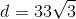## Example Questions

### Example Question #1 : How To Find The Diagonal Of A Cube

Find the length of the diagonal of a cube with side length of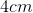.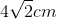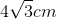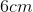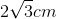Explanation:

We begin with a picture, noting that the diagonal, labeled as, is the length across the cube from one vertex to the opposite side's vertex.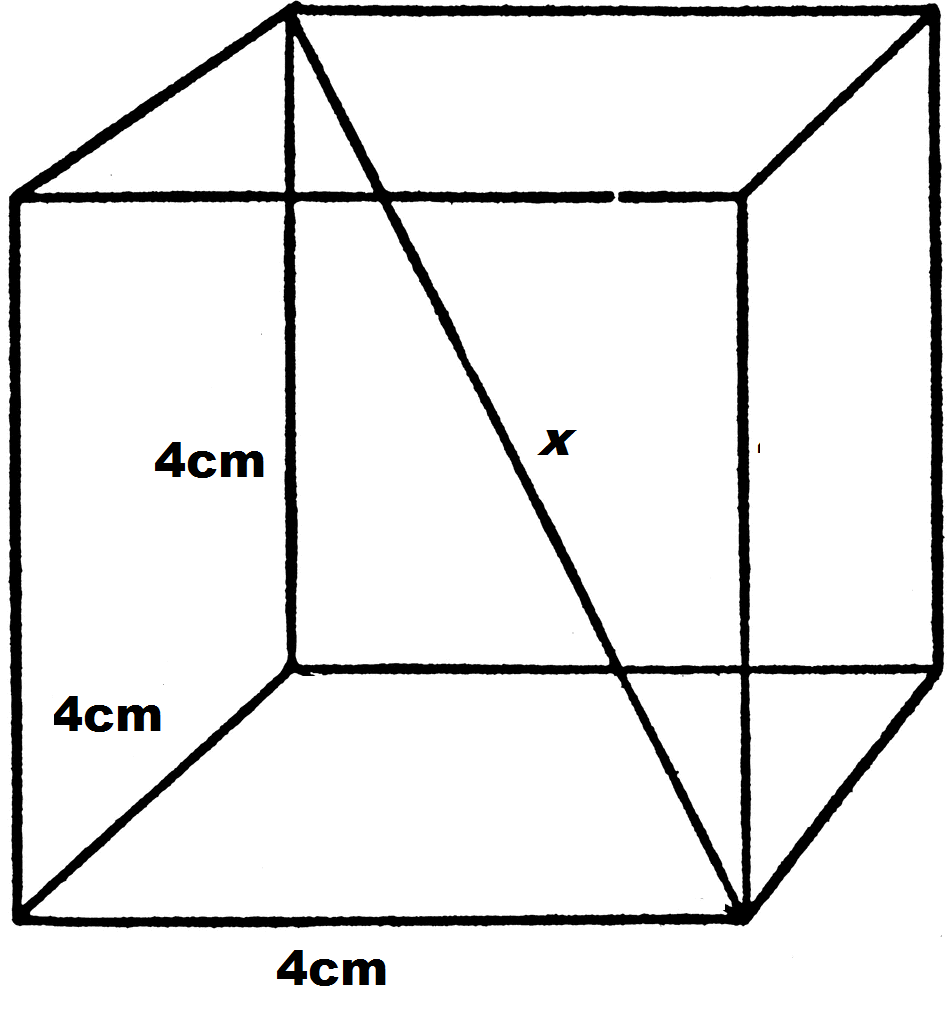However, the trick to solving the problem is to also draw in the diagonal of the bottom face of the cube, which we labeled.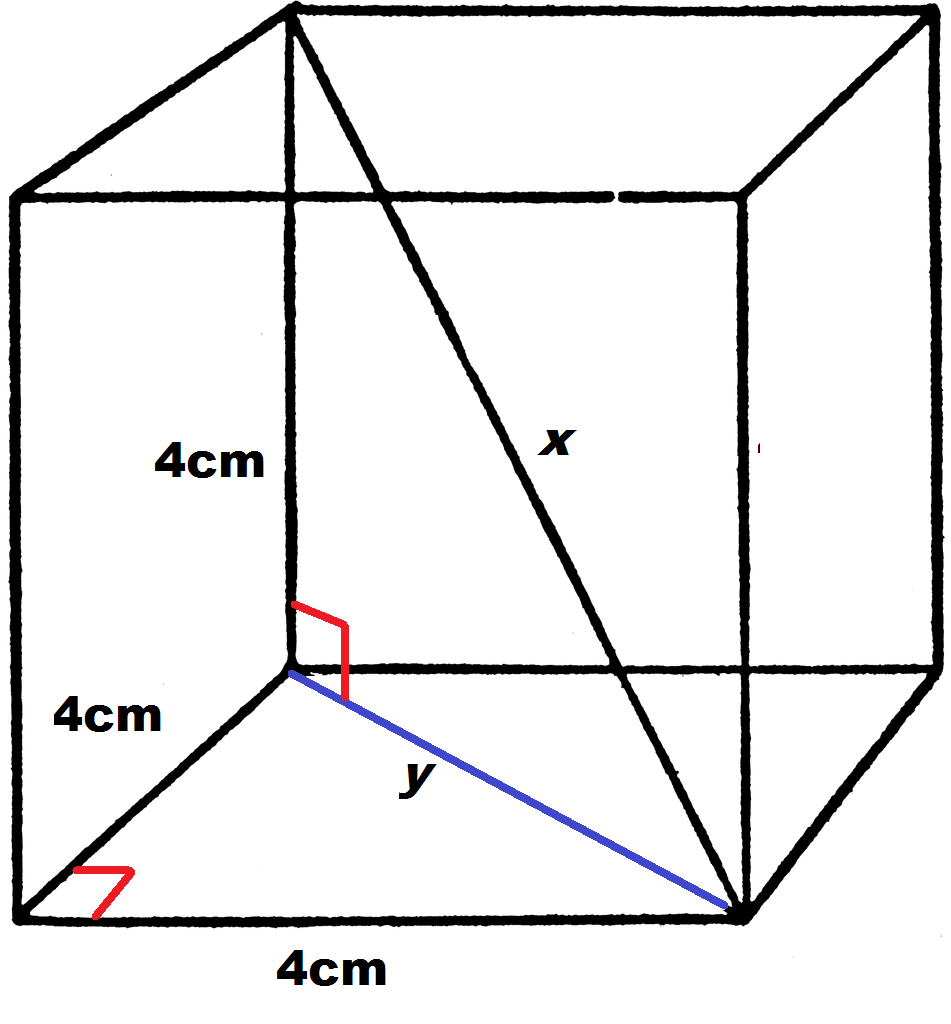Note that this creates two right triangles.  Though our end goal is to find, we can begin by looking at the right triangle in the bottom face to find.  Using either the Pythagorean Theorem or the fact that we have a 45-45-90 right traingle, we can calculate the hypotenuse.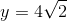Now that we know the value of, we can turn to our second right triangle to findusing the Pythagorean Theorem.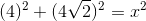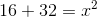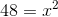Taking the square root of both sides and simplifying gives the answer.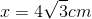### Example Question #2 : How To Find The Diagonal Of A Cube

What is the diagonal length for a cube with volume of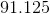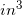?  Round to the nearest hundredth.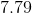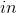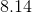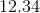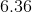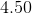Explanation:

Recall that the volume of a cube is computed using the equation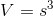, whereis the length of one side of the cube.

So, for our data, we know: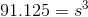Using your calculator, take the cube root of both sides. You can always do this by raisingto thepower if your calculator does not have a varied-root button.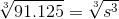If you get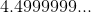, the value really should be rounded up to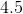. This is because of calculator estimations. So, if the sides are, you can find the diagonal by using a variation on the Pythagorean Theorem working for three dimensions: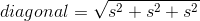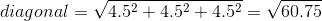This is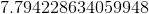. Round it to.

### Example Question #3 : How To Find The Diagonal Of A Cube

What is the length of the diagonal of a cube with a volume of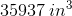?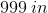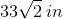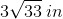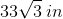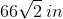Explanation:

Recall that the diagonal of a cube is most easily found when you know that cube's dimensions. For the volume of a cube, the pertinent equation is:, whererepresents the length of one side of the cube. For our data, this gives us: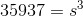Now, you could factor this by hand or use your calculator. You will see thatis.

Now, we find the diagonal by using a three-dimensional version of the Pythagorean Theorem / distance formula: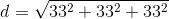or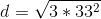You can rewrite this: